# 7 Tips for making your code more ‘pythonic’ and elegant

## 7 use-cases where you can make your python code more nifty, concise, and elegant — without compromising readability.Original image from unsplash.com ( https://unsplash.com/@thisisramiro )

# Use Case 1 :

## When you have to change the type of many of the attributes of your pandas columns / attributes, use a dictionary to do it more elegantly

`df_vin = df_vin.astype(df_box[‘Length’]=df_box[‘Length’].astype(float)df_box[‘Width’]:df_box[‘Width’].astype(float)df_box[‘Height’]: df_box[‘Height’].astype(float)`
`df_vin = df_vin.astype({‘Length’: float,  ‘Width’: int, ‘Height’: float})`

# Use Case 2 :

## When writing a function that returns a value based on a condition, try writing in simple one line conditions for easy readability.

`def is_greater (a,b):    if a > b :         return 1    else:         return 0`
`def is_greater(): return 1 if a > b else 0`
`# Checks if m is an even number is_even=1 if m%2 == 0 else 0`

# Use Case 3:

## When you want to do any list operations, try using List comprehensions.

`list1  = [4,2,3,5,7,8,11,16]`
`list1 = [4,2,3,5,7,8,11,16]list_odd = [] for n in list1 :     if n%2 ==1 :        list_odd.append(n)print(list_odd)    `
`list_odd = [n for n in list1 if n%2 == 1 ]print(list_odd)`

# Use Case 4:

## Use the walrus operator when you want to assign the result of a condition-statement to a Boolean in a nifty manner.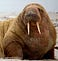Image courtesy : unsplash.com
`my_list = [1,2,3,4,5]`
`if len(my_list) > 3 :     n = Trueelse :     n= Falseprint(n)`
`if n := len(my_list) > 3:    print(n)`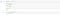using walrus operator v/s traditional way of coding

# Use Case 5:

`lambda arguments: expression`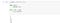traditional way of using a function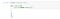pythonic way of executing the same code using a lambda function

# Use Case 6: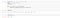making a key value type of list from two different lists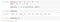using the zip function to combine two lists

# Use Case 7: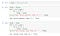Output of the above code

# 4 ways to filter numeric values in dataframes using pandas

Making AI available to everyone. One commit at a time. A.I. M.L| | Quantitative programming | IoT | Python | Arduino | Comp Vision | contact@pythoslabs.com

## More from PythosLabs

Making AI available to everyone. One commit at a time. A.I. M.L| | Quantitative programming | IoT | Python | Arduino | Comp Vision | contact@pythoslabs.com